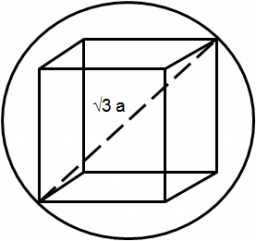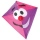# Cube in a sphere

The cube is inscribed in a sphere with volume 7253 cm3. Determine the length of the edges of a cube.

Result

a =  13.9 cm

#### Solution:

$V=7253 \ \text{cm}^3 \ \\ V=\dfrac{ 4 }{ 3 } \pi r^3 \ \\ \ \\ r=\sqrt{ \dfrac{ 3 \cdot \ V }{ 4 \pi } }=\sqrt{ \dfrac{ 3 \cdot \ 7253 }{ 4 \cdot \ 3.1416 } } \doteq 12.0082 \ \text{cm} \ \\ \ \\ D=2 \cdot \ r=2 \cdot \ 12.0082 \doteq 24.0163 \ \text{cm} \ \\ \ \\ u=D=24.0163 \doteq 24.0163 \ \text{cm} \ \\ \ \\ u=\sqrt{ 3 } a \ \\ \ \\ a=u/\sqrt{ 3 }=24.0163/\sqrt{ 3 } \doteq 13.8658 \doteq 13.9 \ \text{cm}$Our examples were largely sent or created by pupils and students themselves. Therefore, we would be pleased if you could send us any errors you found, spelling mistakes, or rephasing the example. Thank you!

Leave us a comment of this math problem and its solution (i.e. if it is still somewhat unclear...):Math student
i am good at this

2 years ago  2 LikesCrazy Butterfly
This is easy.Tips to related online calculators
Need help calculate sum, simplify or multiply fractions? Try our fraction calculator.
Do you want to convert length units?
Tip: Our volume units converter will help you with the conversion of volume units.
Pythagorean theorem is the base for the right triangle calculator.

#### You need to know the following knowledge to solve this word math problem:

We encourage you to watch this tutorial video on this math problem:

## Next similar math problems:

1. Spherical capThe spherical cap has a base radius of 8 cm and a height of 5 cm. Calculate the radius of a sphere of which this spherical cap is cut.
2. An equilateralAn equilateral triangle is inscribed in a square of side 1 unit long so that it has one common vertex with the square. What is the area of the inscribed triangle?
3. EmbankmentThe railway embankment 300 m long has a cross section of an isosceles trapezoid with bases of 14 m and 8 m. The trapezoidal arms are 5 m long. Calculate how much m3 of soil is in the embankment?
4. Circle and squareAn ABCD square with a side length of 100 mm is given. Calculate the radius of the circle that passes through the vertices B, C and the center of the side AD.
5. Concentric circles and chordIn a circle with a diameter d = 10 cm, a chord with a length of 6 cm is constructed. What radius have the concentric circle while touch this chord?Calculate the surface of a quadrilateral pyramid, which has a rectangular base with dimensions a = 8 cm, b = 6 cm and height H = 10 cm.
7. Cone roofHow many m2 of roofing is needed to cover a cone-shaped roof with a diameter of 10 m and a height of 4 m? Add an extra 4% to the overlays.
8. The conicalThe conical candle has a base diameter of 20 cm and a side of 30 cm. How much dm ^ 3 of wax was needed to make it?
9. Diamond area from diagonalsIn the diamond ABCD is AB = 4 dm and the length of the diagonal is 6.4 dm long. What is the area of the diamond?
10. The right triangleThe right triangle ABC has a leg a = 36 cm and an area S = 540 cm2. Calculate the length of the leg b and the median t2 to side b.
11. Wall and body diagonalsThe block/cuboid has dimensions a = 4cm, b = 3cm and c = 12cm. Calculates the length of the wall and body diagonals.
12. Height of pyramidThe pyramid ABCDV has edge lengths: AB = 4, AV = 7. What is its height?
13. Two parallel chordsIn a circle 70 cm in diameter, two parallel chords are drawn so that the center of the circle lies between the chords. Calculate the distance of these chords if one of them is 42 cm long and the second 56 cm.
14. Triangular prismCalculate the surface of a regular triangular prism, the edges of the base are 6 cm long and the height of the prism is 15 cm.
15. FlakesA circle was described on the square, and a semicircle above each side of the square was described. This created 4 "flakes". Which is bigger: the content of the central square or the content of four chips?
16. A kiteChildren have a kite on an 80m long rope, which floats above a place 25m from the place where children stand. How high is the dragon floating above the terrain?
17. RT triangle and heightCalculate the remaining sides of the right triangle if we know side b = 4 cm long and height to side c h = 2.4 cm.